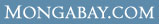WHAT ARE THE AVERAGE Wages & salaries for Computer and mathematical occupations in Rockford, IL ?

 Overview Every year U.S. Department of Labor's Bureau of Labor Statistics releases employment and wage figures. This data was released in May 2006 and covers Wages & salaries for Computer and mathematical occupations in Rockford, IL Computer and mathematical occupations (Occupation [OCC] code: 15-0000) in Rockford, IL (BLS Area code: 40420 ) earned an average wage of \$25.68 per hour and an average annual salary of \$53,420 The BLS estimates that in Rockford, IL there were around 1,300 employed in the field of Computer and mathematical occupations.

Industry Comparison
Industry comparison
Computer and mathematical occupations in Rockford, IL earned 23% less than average for Computer and mathematical occupations in the United States.

Region/area/city comparison
Computer and mathematical occupations in Rockford, IL earned 46% more than the average worker in Rockford, IL.

State salary comparison
Computer and mathematical occupations in Rockford, IL earned 22% more than the average worker in Illinois.

National salary comparison
Computer and mathematical occupations in Rockford, IL earned 36% more than the average worker in the United States.

Wage and salary details
More detailed employment information for Computer and mathematical occupations in Rockford, IL

Hourly wage data for Computer and mathematical occupations in Rockford, IL

 Mean hourly wage: \$25.68 Tenth (10th) percentile hourly wage: \$11.50 Twenty-fifth (25th) percentile hourly wage: \$18.13 Fiftieth (50th) percentile wage [median] hourly wage: \$25.08 Seventy-fifth (75th) percentile hourly wage: \$32.73 Ninetieth (90th) percentile hourly wage: \$40.78

Salary figures for Computer and mathematical occupations in Rockford, IL

 Mean annual salary: \$53,420 Tenth (10th) percentile annual salary: \$23,930 Twenty-fifth (25th) percentile annual salary: \$37,710 Fiftieth (50th) percentile wage [median] annual salary: \$52,160 Seventy-fifth (75th) percentile annual salary: \$68,070 Ninetieth (90th) percentile annual salary: \$84,820

Indexwhat's new | rainforests home | help support the site | madagascar | search | about | contact